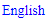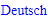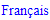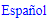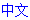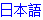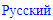Please use logical thinking instead of guessing! Each puzzle only has one solution.

﻿Please use logical thinking instead of guessing! Each puzzle only has one solution.

# Newdoku Rules

## Newdoku Rule 1: Each row and each column consist of different numbers.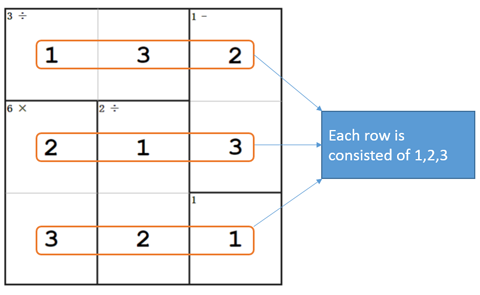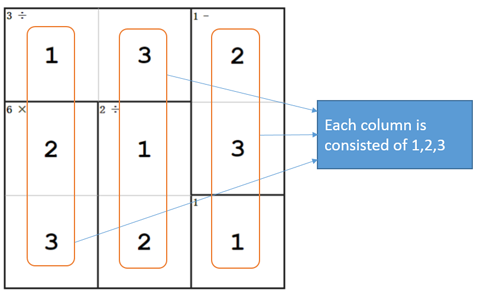In 3x3 questions, each row and each column are consisted of 1, 2,3.
In 4x4 questions, each row and each column are consisted of 1,2,3,4.
As inferred, in 9x9 questions, each row and each column are consisted of 1, 2,3,4,5,6,7,8,9.

## Newdoku Rule 2: Each group of grids has to satisfy required calculation formulas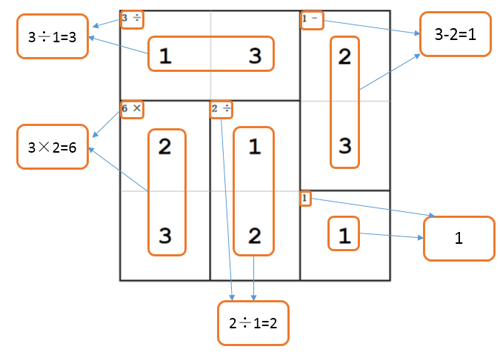Each group of grids has to satisfy required calculation formulas. In Newdoku, there are five different calculation signs. Besides +、-、×、÷, there is another type of group consisted of grids, which do not have calculation signs. At this time, this grid represents number shown on the top-left corner, as shown in following figures.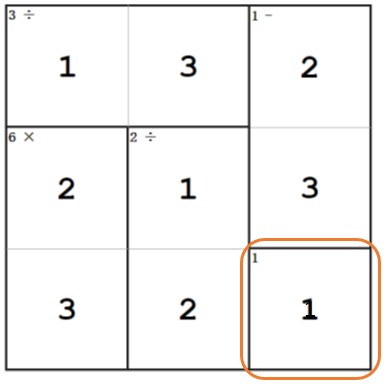The calculation of each group is not related to the sequence.
Numbers of each group can be repetitive. But they have to satisfy rule number one (numbers cannot be repetitive in each column and each row).